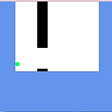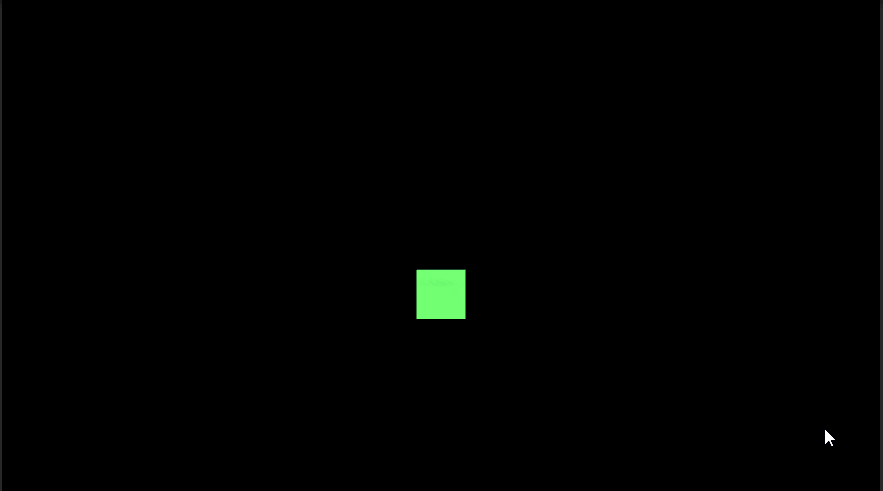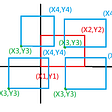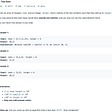# Leetcode Problem Review #1

I’ve decided that I will start reviewing some of the algorithm problems I come across while practicing on LeetCode.

# Problem 1480: Running Sum of 1d Array

Given an array `nums`. We define a running sum of an array as `runningSum[i] = sum(nums…nums[i])`.

Return the running sum of `nums`.

## Example

Input: nums = [1,2,3,4]
Output: [1,3,6,10]
Explanation: Running sum is obtained as follows: [1, 1+2, 1+2+3, 1+2+3+4].

## Constraints

• `1 <= nums.length <= 1000`
• `-10^6 <= nums[i] <= 10^6`
`var runningSum = function(nums){};`

# My Process

## Understanding the Problem

The first thing I did before coding anything was to make sure I understood what the problem was. The examples provided did a pretty good job at explaining what they wanted from me. The input — nums — for this function is an array of random numbers between negative 1 million and positive 1 million and the length of the array is between 1 and 1000 elements. The output should be an array of the running total from each summation of the input elements.

## Breaking it Down

I then started making writing comments in my function about what I need returned and that I need to “do something.” We’ll break down what that “something” is soon.

`var runningSum = function(nums){//DO SOMETHING...//RETURN AN ARRAY THAT HAS THE RUNNING SUM OF THE INPUT ARRAY};`

My thought process was that I needed to have an empty array to begin with so I can push in the sums one by one and then return that array at the end of the function.

`var runningSum = function(nums){   let totalArray = []//DO SOMETHING...//RETURN AN ARRAY THAT HAS THE RUNNING SUM OF THE INPUT ARRAY   return totalArray};`

Now about the “do something” comment. I felt like I should use a for loop through each element in the nums input array, that way I could do some kind of summation while working on one element at a time. I will expand in this soon.

`var runningSum = function(nums){   let totalArray = []   for (let i = 0; i < nums.length; i++ ){      //DO SOMETHING IN LOOP...   }//RETURN AN ARRAY THAT HAS THE RUNNING SUM OF THE INPUT ARRAY   return totalArray};`

So now that at this point I started to think about how I would push a running sum of numbers into totalArray. I can see how running sums work by the examples provided. If our input is [1,2,3,4] our output is [1,3,6,10] because…

`current sum | current element | new sum (pushed into array)----------------------------------------------------------     0      |        1        |    1     1      |        2        |    3     3      |        3        |    6     6      |        4        |    10`

So in our function I’m going to create a variable called currentSum and set that to 0 as the starting point since we haven’t started adding anything yet. Then as the function goes through the for loop, I’m going to add the current element to the currentSum variable and push that new sum into the totalArray variable.

`var runningSum = function(nums){   let totalArray = []//SET currentSum VARIABLE TO 0   let currentSum = 0   for (let i = 0; i < nums.length; i++ ){      //ADD THE CURRENT ELEMENT TO currentSum      currentSum += nums[i]      //PUSH THE currentSum INTO TOTAL ARRAY AFTER THE CURRENT    ELEMENT IS ADDED TO currentSum      totalArray.push(currentSum)   }//RETURN AN ARRAY THAT HAS THE RUNNING SUM OF THE INPUT ARRAY   return totalArray};`

## SOLVED

`var runningSum = function(nums){   let totalArray = []   let currentSum = 0   for (let i = 0; i < nums.length; i++ ){      currentSum += nums[i]      totalArray.push(currentSum)   }   return totalArray};`

# My Thoughts…

It took me some time to complete this problem. I overthought a lot of things the first time I did this problem because I did not fully understand the problem. I overthought everything so much that I was about to put a loop inside of a loop with O notation of O(n²) which would be ridiculous for a simple problem like this. That’s when I stopped and started over from scratch.

I learned it’s best to make sure you 100% what is being asked of you and to pseudocode everything you can and work using a simple input that makes more sense to you. After you’re able to make that work, expand and try using a more complex input.

I’m hoping that sharing my detailed problem-solving process helps someone form their own process that works great with them.

--

--

--

## More from Ajak Cyer

Software Engineering Student at Flatiron NYC. Learning day by day 💻

Love podcasts or audiobooks? Learn on the go with our new app.

## Ionic 5 Charts & Graphs using Chart.js Library## JavaScript Project## Real-time chat room with Larasocket, Tailwind, Alpine, Livewire, and Laravel## How To Use Highlight.js## Generics in Typescript — How To Type Reusable Parts of Code?## Generate a self-contained executable file with .net core 3.1 & VS2019## Inheritance and Required Component## Ajak Cyer

Software Engineering Student at Flatiron NYC. Learning day by day 💻

## The Blind 75 Leetcode Series: Maximum Subarray## 836. Rectangle Overlap## 1 — Two Sum